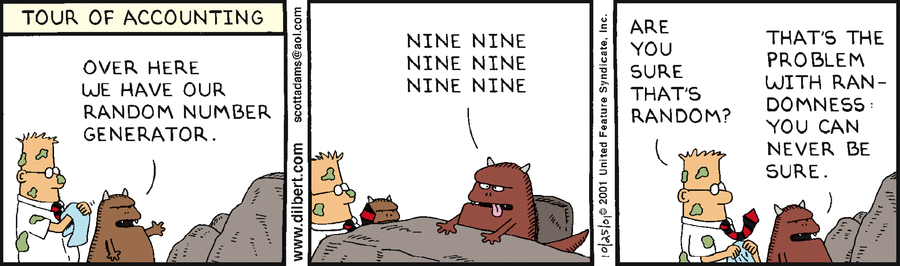# Process - (Stochastic|random) process

A stochastic process is a process where the variable is a random variable that is time dependent.

A stochastic process is not deterministic, ie given a particular input, he is: * more likely to produce some outputs * less likely to produce others.

## Example

Example of random variable:

• number of concurrent user
• number of jobs in a queue
• internet traffic
• system available capacity
• stock price
• air temperatur

## Documentation / Reference

• Georgii, H.-O. 2002. Stochastik. DeGruyter.

Discover MoreNumber - Random (Stochastic|Independent) or (Balanced)

Think of randomness as a lack of pattern. Something random should be unpredictable. We shouldn’t be able to predict the next value of the sequence The degree to which a system has no pattern is known...Process - (System) Simulation

process simulation. The basic model components are queues, (Queue theory to model finite resources) stochastic distributions (based on random number streams) to model the time Each collect...Process - Poisson Process

The Poisson process is a stochastic process in which events occur: continuously independently (of the time since the last event) - (ie random) at a constant / known average rate in a fixed interval...Soft Skill - Bias

PareidoliaPareidolia is the tendency to interpret a vague stimulus as something known to the observer, such as seeing shapes in clouds, seeing faces in inanimate objects or abstract patterns, or hearing...Statistics - Random Variable (Random quantity|Aleatory variable|Stochastic variable)

Random variable is also known as: random quantity, aleatory variable, or stochastic variable A random variable represents the result of a random process. The random variable value is the summary...
Time Series (Collection of time data)

A time series is sequential collection of data measured one by one: at fixed time intervals (for instance computer monitoring) or when it happens (for a business process or application) A time-series...